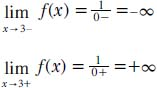NextPrevious

# How is infinity treated when discussing limits?

Defining infinity is a definite part of calculus, especially when discussing limits and “negative” and “positive” infinity. Whenever the inverse of a small number is taken, a large number is generated, and vice versa. In calculus, this is written as:

But ±∞ are no ordinary numbers, because they do not obey the usual rules of arithmetic, such as ∞ + 1 = ∞; ∞ - 1 = ∞; 2 × ∞ = ∞; and so on. Therefore, in calculus functions, and thus limits, infinity is treated much differently. For example, for the function f(x) = 1/x - 3, when x → 3, then x - 3 → 0. The limit function then becomes:This example can be seen in the accompanying graph.

Close

This is a web preview of the "The Handy Math Answer Book" app. Many features only work on your mobile device. If you like what you see, we hope you will consider buying. Get the App